SSC CGL Previous Year Questions: Profit & Loss - 5

# SSC CGL Previous Year Questions: Profit & Loss - 5 - SSC CGL

Test Description

## 22 Questions MCQ Test SSC CGL (Tier - 1) - Previous Year Papers (Topic Wise) - SSC CGL Previous Year Questions: Profit & Loss - 5

SSC CGL Previous Year Questions: Profit & Loss - 5 for SSC CGL 2023 is part of SSC CGL (Tier - 1) - Previous Year Papers (Topic Wise) preparation. The SSC CGL Previous Year Questions: Profit & Loss - 5 questions and answers have been prepared according to the SSC CGL exam syllabus.The SSC CGL Previous Year Questions: Profit & Loss - 5 MCQs are made for SSC CGL 2023 Exam. Find important definitions, questions, notes, meanings, examples, exercises, MCQs and online tests for SSC CGL Previous Year Questions: Profit & Loss - 5 below.
Solutions of SSC CGL Previous Year Questions: Profit & Loss - 5 questions in English are available as part of our SSC CGL (Tier - 1) - Previous Year Papers (Topic Wise) for SSC CGL & SSC CGL Previous Year Questions: Profit & Loss - 5 solutions in Hindi for SSC CGL (Tier - 1) - Previous Year Papers (Topic Wise) course. Download more important topics, notes, lectures and mock test series for SSC CGL Exam by signing up for free. Attempt SSC CGL Previous Year Questions: Profit & Loss - 5 | 22 questions in 23 minutes | Mock test for SSC CGL preparation | Free important questions MCQ to study SSC CGL (Tier - 1) - Previous Year Papers (Topic Wise) for SSC CGL Exam | Download free PDF with solutions
 1 Crore+ students have signed up on EduRev. Have you?
SSC CGL Previous Year Questions: Profit & Loss - 5 - Question 1

### A reduction of 20% in the price of sugar enables me to purchase 5 kg more for ₹ 600. Find the price of sugar per kg before reduction of price.         (SSC CGL 1st Sit. 2011)

Detailed Solution for SSC CGL Previous Year Questions: Profit & Loss - 5 - Question 1

Let CP = x, Total ₹ = 600, Sugar bought = 600/x
ATQ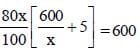480 + 4x = 600
4x = 120
x = 30

SSC CGL Previous Year Questions: Profit & Loss - 5 - Question 2

### 20% loss on selling price is what per cent loss on the cost price?         (SSC CGL 1st Sit. 2011)

Detailed Solution for SSC CGL Previous Year Questions: Profit & Loss - 5 - Question 2

Let SP = 100
Loss% on SP = 20%
CP = 100 + 20 = 120
Loss % of CP =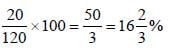SSC CGL Previous Year Questions: Profit & Loss - 5 - Question 3

### X sells two articles for ₹ 4,000 each with no loss and no gain in the interaction. If one was sold at a gain of 25% the other is sold at a loss of       (SSC CGL 1st Sit. 2011)

Detailed Solution for SSC CGL Previous Year Questions: Profit & Loss - 5 - Question 3

Cost price of first article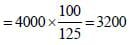Total cost price of both articles = Total selling price of both articles = 4000 + 4000 = 8000
Cost price of second articles = 8000 – 3200 = 4800
Selling price of second articles = 4000
Loss on second articles = 4800 – 4000 = 800
Required % =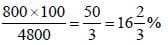SSC CGL Previous Year Questions: Profit & Loss - 5 - Question 4

If the price of sugar is raised by 25%, find by how much percent a householder must reduce his consumption of sugar so as not to increase his expenditure?        (SSC CGL 1st Sit. 2011)

Detailed Solution for SSC CGL Previous Year Questions: Profit & Loss - 5 - Question 4

Percentage decrease = (25/125) x 100 = 20

SSC CGL Previous Year Questions: Profit & Loss - 5 - Question 5

When the price of sugar decreases by 10%, a man could buy 1 kg more for ₹ 270. Then the original price of sugar per kg is        (SSC CGL 1st Sit. 2011)

Detailed Solution for SSC CGL Previous Year Questions: Profit & Loss - 5 - Question 5

Let the original price of sugar be ₹ x/kg.
∴ New price = ₹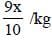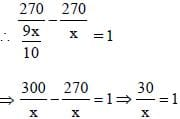⇒ x = ₹ 30/kg

SSC CGL Previous Year Questions: Profit & Loss - 5 - Question 6

The cost price of an article is 40% of the selling price. What percent of the cost price is the selling price?         (SSC CGL 1st Sit. 2011)

Detailed Solution for SSC CGL Previous Year Questions: Profit & Loss - 5 - Question 6

Let the S.P. of the article = ₹ 100
∴ C.P. = ₹ 40
∴ Required percentage =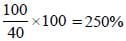SSC CGL Previous Year Questions: Profit & Loss - 5 - Question 7

A trader bought two horses for ₹19,500. He sold one at a loss of 20% and the other at a profit of 15%. If the selling price of each horse is the same, then their cost prices are respectively.        (SSC CGL 1st Sit. 2011)

Detailed Solution for SSC CGL Previous Year Questions: Profit & Loss - 5 - Question 7

The sum of cost prices of two horses is ₹ x. One of them is sold at a loss of a% and other is sold at a gain of b% and their S.P. is same.
∴ C.P. of horse sold at a loss of a%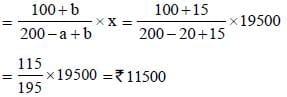∴ C.P. of second article = ₹ 8000.

SSC CGL Previous Year Questions: Profit & Loss - 5 - Question 8

Krishna purchased a number of articles at ₹10 for each and the same number for ₹ 14 each. He mixed them together and sold them for ₹13 each. Then his gain or loss percent is        (SSC CGL 1st Sit. 2011)

Detailed Solution for SSC CGL Previous Year Questions: Profit & Loss - 5 - Question 8

Average cost of =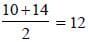QP = 13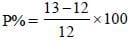= 8(1/3)

SSC CGL Previous Year Questions: Profit & Loss - 5 - Question 9

While selling a watch, a shopkeeper gives a discount of 5%. If he gives a discount of 6%, he earns ₹ 15 less as profit. What is the marked price of the watch?        (SSC CGL 1st Sit. 2011)

Detailed Solution for SSC CGL Previous Year Questions: Profit & Loss - 5 - Question 9

Difference in discount = 1%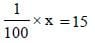x = 1500

SSC CGL Previous Year Questions: Profit & Loss - 5 - Question 10

If the selling price of 10 articles is equal to the cost price of 11 articles, then the gain percent is        (SSC CGL 1st Sit. 2011)

Detailed Solution for SSC CGL Previous Year Questions: Profit & Loss - 5 - Question 10

Let the C.P. of each article be ₹ 1.
∴ C.P. of articles = ₹ 10
and S.P. of 10 articles = ₹ 11
∴ Profit percent =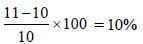SSC CGL Previous Year Questions: Profit & Loss - 5 - Question 11

What single discount is equivalent to two successive discounts of 20% and 15%?        (SSC CGL 1st Sit. 2011)

Detailed Solution for SSC CGL Previous Year Questions: Profit & Loss - 5 - Question 11

Single equivalent discount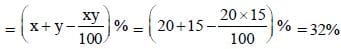SSC CGL Previous Year Questions: Profit & Loss - 5 - Question 12

A shopkeeper allows a discount of 10% to his customers and still gains. 20%. Find the marked price of the article which costs ₹ 450.       (SSC CGL 1st Sit. 2011)

Detailed Solution for SSC CGL Previous Year Questions: Profit & Loss - 5 - Question 12

Let the marked price of the article be ₹ x.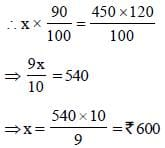SSC CGL Previous Year Questions: Profit & Loss - 5 - Question 13

The price of an article was first increased by 10% and then again by 20%. If the last increased price be ₹ 33, the original price was      (SSC CGL 2nd Sit. 2010)

Detailed Solution for SSC CGL Previous Year Questions: Profit & Loss - 5 - Question 13

Net increase percentage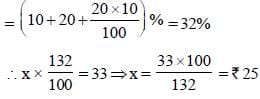SSC CGL Previous Year Questions: Profit & Loss - 5 - Question 14

If on a marked price, the difference of selling prices with a discount of 30% and two successive discounts of 20% and 10% is ₹72, then the marked price (in rupees) is        (SSC CGL 2nd Sit. 2010)

Detailed Solution for SSC CGL Previous Year Questions: Profit & Loss - 5 - Question 14

Let the marked price be ₹ x.
∴ In case I, SP = ₹ (70x/100)
Single discount equivalent to successive discounts of 20% and 10%.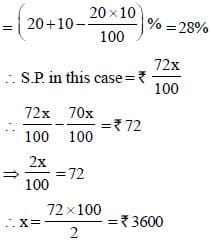SSC CGL Previous Year Questions: Profit & Loss - 5 - Question 15

If an article is sold at 200% profit, then the ratio of its cost price to its selling price will be       (SSC CGL 1st Sit. 2010)

Detailed Solution for SSC CGL Previous Year Questions: Profit & Loss - 5 - Question 15

Let CP = 100
P = 200
SP = CP + P = 300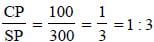SSC CGL Previous Year Questions: Profit & Loss - 5 - Question 16

If the cost price of 15 books is equal to the selling price of 20 books, the loss percent is      (SSC CGL 1st Sit. 2010)

Detailed Solution for SSC CGL Previous Year Questions: Profit & Loss - 5 - Question 16

If the CP of each book be ₹1, then
SP of 20 books = ₹15
CP of 20 books = ₹20SSC CGL Previous Year Questions: Profit & Loss - 5 - Question 17

If there is a profit of 20% on the cost price of an article, the percentage of profit calculated on its selling price will be     (SSC CGL 1st Sit. 2010)

Detailed Solution for SSC CGL Previous Year Questions: Profit & Loss - 5 - Question 17

If the CP = ₹100, then SP = ₹120 and gain = ₹ 20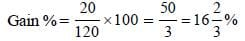SSC CGL Previous Year Questions: Profit & Loss - 5 - Question 18

By selling an article, a man makes a profit of 25% of its selling price. His profit percent is       (SSC CGL 2nd Sit. 2010)

Detailed Solution for SSC CGL Previous Year Questions: Profit & Loss - 5 - Question 18

If the S.P. of article be ₹x, then its CP =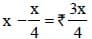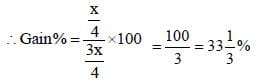SSC CGL Previous Year Questions: Profit & Loss - 5 - Question 19

By selling a bicycle for ₹ 2,850, Aa shopkeeper gains 14%. If the profit is reduced to 8%, then the selling price will be      (SSC CGL 2nd Sit. 2010)

Detailed Solution for SSC CGL Previous Year Questions: Profit & Loss - 5 - Question 19

C.P. of bicycle = (100/114) x 2850 = ₹2500
S.P. for a profit of 8%= (108/100) x 2500 = ₹2700

SSC CGL Previous Year Questions: Profit & Loss - 5 - Question 20

A shopkeeper earns a profit of 12% on selling a book at 10% discount on the printed price. The ratio for the cost price and the printed price of the book is       (SSC CGL 1st Sit. 2010)

Detailed Solution for SSC CGL Previous Year Questions: Profit & Loss - 5 - Question 20

Let the CP be ₹100.
∴ SP = ₹112
If the marked price be ₹x, then
90% of x = 112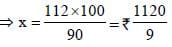∴ Required ratio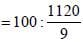= 900 : 1120 = 45 : 56

SSC CGL Previous Year Questions: Profit & Loss - 5 - Question 21

A manufacturer marked an article at ₹50 and sold it allowing 20% discount. If his profit was 25% then the cost price of the article was      (SSC CGL 1st Sit. 2010)

Detailed Solution for SSC CGL Previous Year Questions: Profit & Loss - 5 - Question 21

Marked price = ₹50
S.P. after discount = 80% of 50 = ₹40
If the CP of article be `x, then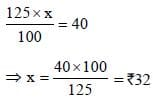SSC CGL Previous Year Questions: Profit & Loss - 5 - Question 22

If the ratio of cost price and selling price of an article be as 10 : 11, the percentage of profit is      (SSC CGL 1st Sit. 2010)

Detailed Solution for SSC CGL Previous Year Questions: Profit & Loss - 5 - Question 22

Gain = 11x – 10x = ₹x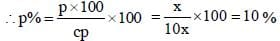## SSC CGL (Tier - 1) - Previous Year Papers (Topic Wise)

250 tests
Information about SSC CGL Previous Year Questions: Profit & Loss - 5 Page
In this test you can find the Exam questions for SSC CGL Previous Year Questions: Profit & Loss - 5 solved & explained in the simplest way possible. Besides giving Questions and answers for SSC CGL Previous Year Questions: Profit & Loss - 5, EduRev gives you an ample number of Online tests for practice

250 tests